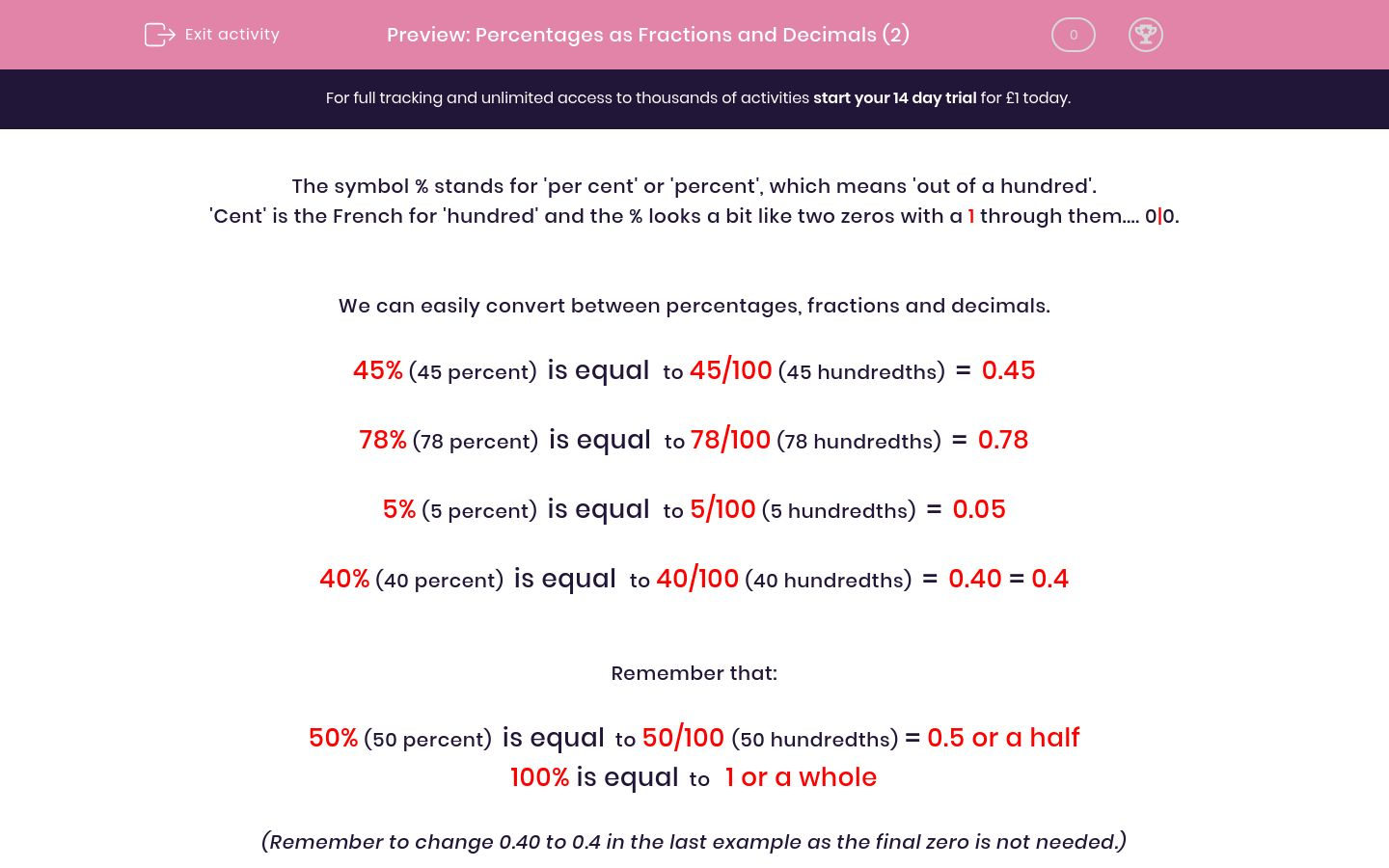# Percentages as Fractions and Decimals (2)

In this worksheet, students recognise the percent symbol (%) and understand that percent relates to ‘number of parts per hundred’, writing percentages as fractions with denominator 100, and as a decimal.Key stage:  KS 2

Curriculum topic:   Maths and Numerical Reasoning

Curriculum subtopic:   Fractions

Difficulty level:### QUESTION 1 of 10

The symbol % stands for 'per cent' or 'percent', which means 'out of a hundred'.

'Cent' is the French for 'hundred' and the % looks a bit like two zeros with a 1 through them.... 0|0.

We can easily convert between percentages, fractions and decimals.

45% (45 percent)  is equal  to 45/100 (45 hundredths)  =  0.45

78% (78 percent)  is equal  to 78/100 (78 hundredths)  =  0.78

5% (5 percent)  is equal  to 5/100 (5 hundredths)  =  0.05

40% (40 percent)  is equal  to 40/100 (40 hundredths)  =  0.40 = 0.4

Remember that:

50% (50 percent)  is equal  to 50/100 (50 hundredths) = 0.5 or a half

100% is equal  to   1 or a whole

(Remember to change 0.40 to 0.4 in the last example as the final zero is not needed.)

Select all the answers which are equal to:

25%

2%

25/100

0.25

25/10

2.5

Select all the answers which are equal to:

64%

60%

6/100

64/100

64 hundredths

0.64

Select all the answers which are equal to:

10%

a half

10/100

one hundredth

0.10

0.1

Select all the answers which are equal to:

2%

1/2

2/100

two hundredths

0.2

0.02

Select all the answers which are equal to:

50%

1/2

50/100

fifty hundredths

0.5

0.50

Select all the answers which are equal to:

79%

80%

80/100

7.9

79/100

0.79

Select all the answers which are equal to:

90%

a ninth

90/100

90 hundredths

0.9

0.09

Select all the answers which are equal to:

9%

1/9

9/100

nine hundredths

0.9

0.09

Select all the answers which are equal to:

99%

1/99

99/100

ninety-nine hundredths

0.99

99

Select all the answers which are equal to:

100%

1/100

100/100

a whole

1

100

• Question 1

Select all the answers which are equal to:

25%

25/100
0.25
EDDIE SAYS
25% = 25 hundredths = 0.25
• Question 2

Select all the answers which are equal to:

64%

64/100
64 hundredths
0.64
EDDIE SAYS
64% = 64 hundredths = 0.64
• Question 3

Select all the answers which are equal to:

10%

10/100
0.10
0.1
EDDIE SAYS
10% = 10 hundredths = 0.10 = 0.1
• Question 4

Select all the answers which are equal to:

2%

2/100
two hundredths
0.02
EDDIE SAYS
2% = 2 hundredths = 0.02
• Question 5

Select all the answers which are equal to:

50%

1/2
50/100
fifty hundredths
0.5
0.50
EDDIE SAYS
50% = 50 hundredths = ½ = 0.50 = 0.5
• Question 6

Select all the answers which are equal to:

79%

79/100
0.79
EDDIE SAYS
79% = 79 hundredths = 0.79
• Question 7

Select all the answers which are equal to:

90%

90/100
90 hundredths
0.9
EDDIE SAYS
90% = 90 hundredths = 0.90 = 0.9
• Question 8

Select all the answers which are equal to:

9%

9/100
nine hundredths
0.09
EDDIE SAYS
9% = 9 hundredths = 0.09
• Question 9

Select all the answers which are equal to:

99%

99/100
ninety-nine hundredths
0.99
EDDIE SAYS
99% = 99 hundredths = 0.99
• Question 10

Select all the answers which are equal to:

100%

100/100
a whole
1
EDDIE SAYS
100% = 100 hundredths = 1.00 = 1 = a whole
---- OR ----